# An Introduction to synlik

## Introduction

The synlik package provides Synthetic Likelihood methods for intractable likelihoods. The package is meant to be as general purpose as possible: as long as you are able to simulate data from your model you should be able to fit it.

## Creating a synlik object

A synlik object is mainly composed of the simulator, which is the function that simulates data from the model of interest. In addition, it is possible to specify a function summaries, which transforms the data generated by simulator into summary statistics. The simulator can generate any kind of output, as long as summaries is able to transform it into a matrix where each row is a simulated vector of statistics. If summaries is not specified, then simulator has to output such a matrix.

Here we set-up a synlik object representing the Ricker map. The observations are given by $$Y_t \sim Pois(\phi N_t)$$, where the hidden state has the following dynamics: $$N_t = r N_{t-1} exp( -N_{t-1} + e_t )$$. This is how we create the object:

library(synlik)

## Loading required package: Rcpp

ricker_sl <- synlik(simulator = rickerSimul,
summaries = rickerStats,
param = c( logR = 3.8, logSigma = log(0.3), logPhi = log(10) ),
extraArgs = list("nObs" = 50, "nBurn" = 50)
)


Here:

• rickerSimul and rickerStats are functions provided by synlik.
• param is a named vector that contains the log-parameters that will be used by rickerSimul(param, nsim, extraArgs, ...).
• extraArgs contains additional arguments required by rickerSimul, see ?rickerSimul for details.

Now we are ready to simulate data from the object:

ricker_sl@data <- simulate(ricker_sl, nsim = 1, seed = 54)


Here ricker_sl@data is just a vector, but synlik allows the simulator to simulate any kind of object, so it is often necessary encapsulate an adequate plotting function into the object:

ricker_sl@plotFun <- function(input, ...) plot(drop(input), type = 'l', ylab = "Pop", xlab = "Time", ...)
plot(ricker_sl)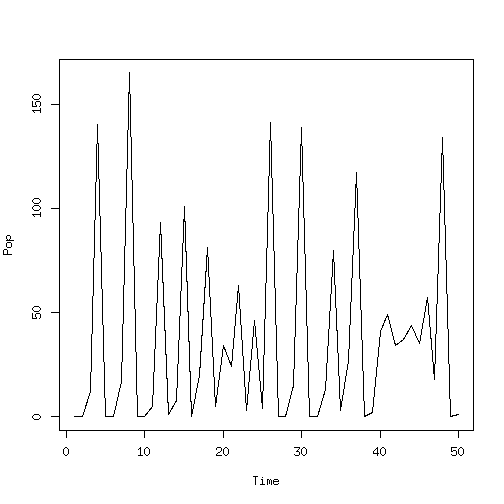If we want to simulate several datasets we simply do:

tmp <- simulate(ricker_sl, nsim = 10)
dim(tmp)

##  10 50


So far we have just simulated data, not summary statistics. In this particular example rickerStats needs to be passed the reference data in ricker_sl@data in order to be able to calculate the statistics. We can do that by using the slot extraArgs:

ricker_sl@extraArgs$obsData <- ricker_sl@data  Now we are ready to simulate summary statistics: tmp <- simulate(ricker_sl, nsim = 2, stats = TRUE) tmp  ## [,1] [,2] [,3] [,4] [,5] [,6] [,7] ## [1,] 0.8016316 0.0005366660 2.427694e-05 0.03743647 -0.2248205 36.90 17 ## [2,] 0.9022046 0.0003317784 1.959014e-05 0.05564859 -0.2073172 37.38 21 ## [,8] [,9] [,10] [,11] [,12] [,13] ## [1,] 3180.570 -903.5390 -370.0129 -526.6177 46.91435 -203.1856 ## [2,] 3555.596 -863.9911 -930.2048 547.7001 -842.12995 225.4435  and to check their approximate normality: checkNorm(ricker_sl)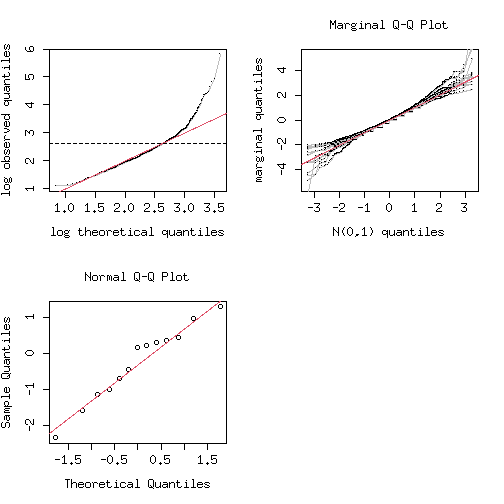## Looking at the synthetic likelihood If we want to estimate the value of the synthetic likelihood at a certain location in the parameter space, we can do it by using the function slik as follows: slik(ricker_sl, param = c(logR = 3.8, logSigma = log(0.3), logPhi = log(10)), nsim = 1e3)  ##  -20.44001  We can also look at slices of this function wrt each parameter: slice(object = ricker_sl, ranges = list("logR" = seq(3.5, 3.9, by = 0.01), "logPhi" = seq(2, 2.6, by = 0.01), "logSigma" = seq(-2, -0.5, by = 0.02)), param = c(logR = 3.8, logSigma = log(0.3), logPhi = log(10)), nsim = 1000)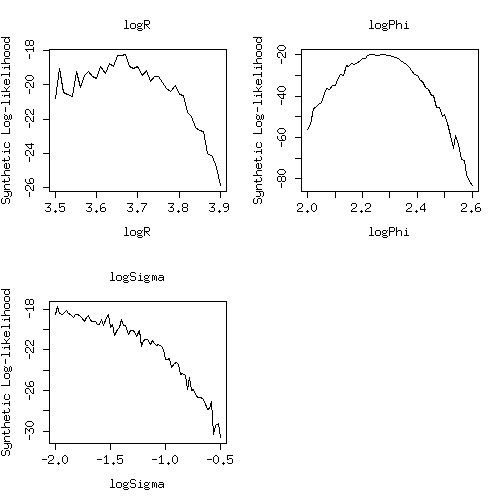Finally we can have 2D slices with respect to pairs of parameters: slice(object = ricker_sl, ranges = list("logR" = seq(3.5, 3.9, by = 0.02), "logPhi" = seq(2, 2.6, by = 0.02)), pairs = TRUE, param = c(logR = 3.8, logSigma = log(0.3), logPhi = log(10)), nsim = 1000, multicore = TRUE, ncores = 2)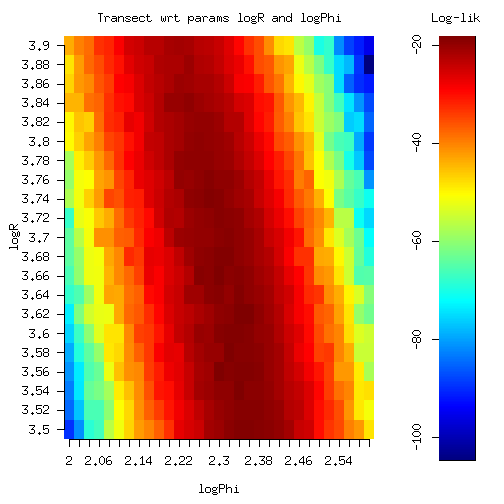Notice that here we have used the multicore option, which distributes the computation among different cores or cluster nodes. Also slik provides this option, but for such a simple model the time needed to set up the cluster is longer than the simulation time. ## Estimating the parameters by MCMC The unknown model parameters can be estimated by MCMC, using the smcmc function. Here is an example (you might want to increase niter): ricker_sl <- smcmc(ricker_sl, initPar = c(3.2, -1, 2.6), niter = 10, burn = 3, priorFun = function(input, ...) sum(input), propCov = diag(c(0.1, 0.1, 0.1))^2, nsim = 500)  Notice that priorFun returns the log-density of the prior. If we have not reached convergence we can do some more MCMC iterations by using the continue generic: ricker_sl <- continue(ricker_sl, niter = 10)  Finally we can plot the MCMC output (here we plot a pre-computed object): data(ricker_smcmc) addline1 <- function(parNam, ...) abline(h = ricker_smcmc@param[parNam], lwd = 2, lty = 2, col = 3) addline2 <- function(parNam, ...) abline(v = ricker_smcmc@param[parNam], lwd = 2, lty = 2, col = 3) plot(ricker_smcmc, addPlot1 = "addline1", addPlot2 = "addline2")  ##  "Plotting the MCMC chains"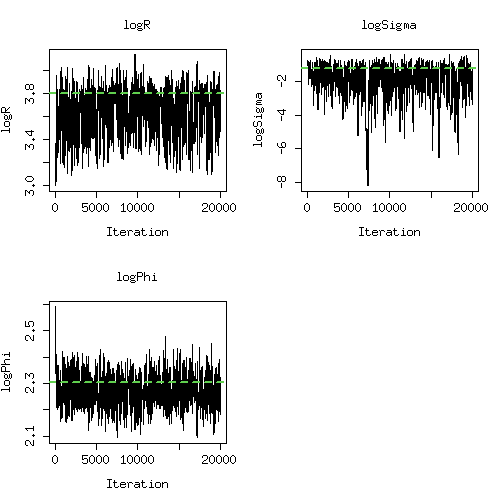## Press <Enter> to see the next plot... ##  "The posterior densities"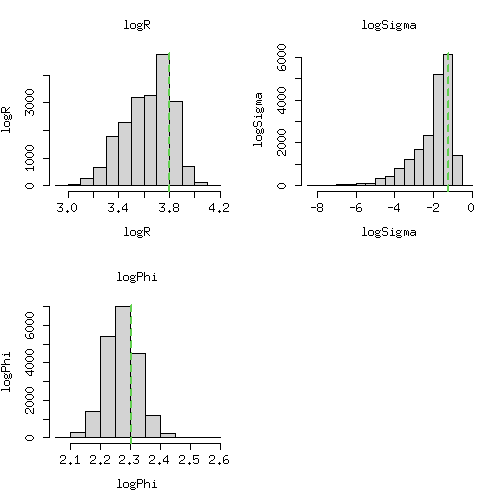## Press <Enter> to see the next plot... ##  "Plotting the log-likelihood chain"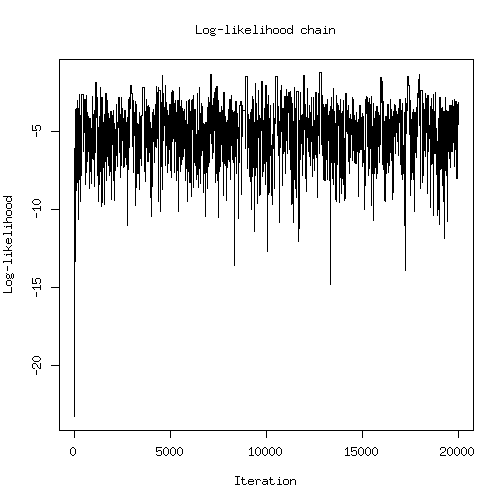## Press <Enter> to see the next plot... ##  "Plotting correlation structure of the posterior sample"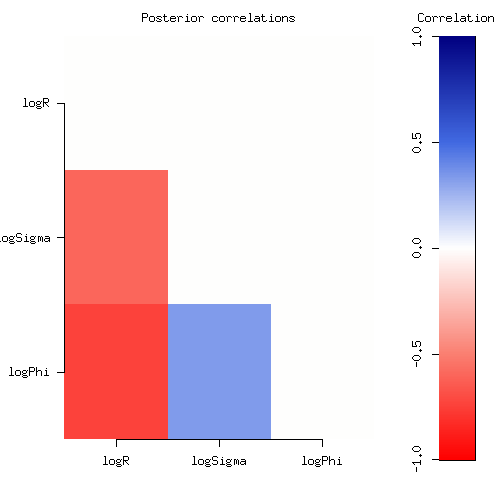## Press <Enter> to see the next plot...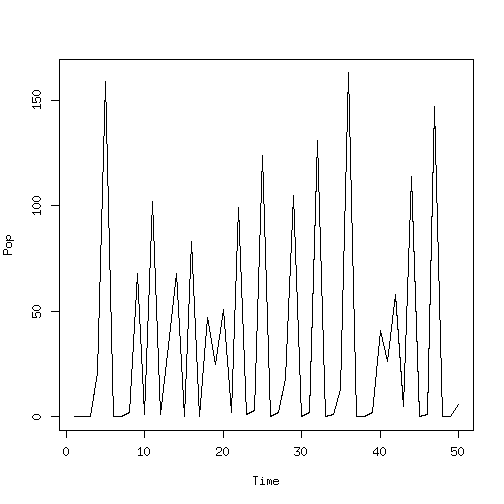were we have added the green dotted lines, indicating the position of the true parameters. ## Blowfly example As a more challenging example, let us consider the Blowfly model proposed by Wood (2010): $N_{t} = R_t + S_t,$ where $R_t \sim \text{Pois}(PN_{t-\tau}e^{-\frac{N_{t-\tau}}{N_0}}e_t),$ represents delayed recruitment process, while: $S_t \sim \text{binom}(e^{-\delta\epsilon_t}, N_{t-1}),$ denotes the adult survival process. Finally $$e_t$$ and $$\epsilon_t$$ are independent gamma distributed random variables, with unit means and variances equal to $$\sigma_p^2$$ and $$\sigma_d^2$$ respectively. We start by creating a synlik object: blow_sl <- synlik(simulator = blowSimul, summaries = blowStats, param = log( c( "delta" = 0.16, "P" = 6.5, "N0" = 400, "var.p" = 0.1, "tau" = 14, "var.d" = 0.1) ), extraArgs = list("nObs" = 200, "nBurn" = 200, "steps" = 1), plotFun = function(input, ...){ plot(drop(input), type = 'l', ylab = "Pop", xlab = "Time", ...) } )  for more details see ?blow_sl. As before we simulate data and we store it back into the object: blow_sl@data <- simulate(blow_sl, seed = 84) blow_sl@extraArgs$obsData <- blow_sl@data


As for the Ricker example, blowStats needs the observed data to calculate the statistics, hence we store it into extraArgs$obsData. Notice that this is just a peculiarity of the chosen summaries function. Then, we can estimate the parameters by adaptive MCMC (you might want to increase niter): blow_sl <- smcmc(blow_sl, initPar = log( c( "delta" = 0.1, "P" = 8, "N0" = 600, "sig.p" = 0.2, "tau" = 17, "sig.d" = 0.3) ), niter = 2, burn = 0, propCov = diag(rep(0.001, 6)), nsim = 500, prior = function(input, ...){ sum(input) + dunif(input, log(0.01), log(1), log = TRUE) + dunif(input, log(0.01), log(1), log = TRUE) }, targetRate = 0.15, multicore = FALSE )  We plot the results on the original scale (here we plot a pre-computed object): data(blow_smcmc) tmpTrans <- rep("exp", 6) names(tmpTrans) <- names(blow_smcmc@param) plot(blow_smcmc, trans = tmpTrans)  ##  "Plotting the MCMC chains"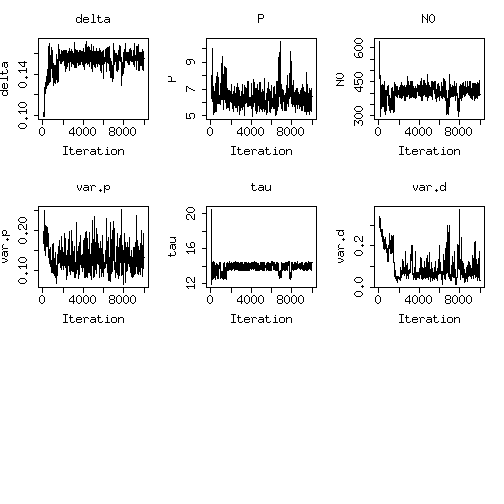## Press <Enter> to see the next plot... ##  "The posterior densities"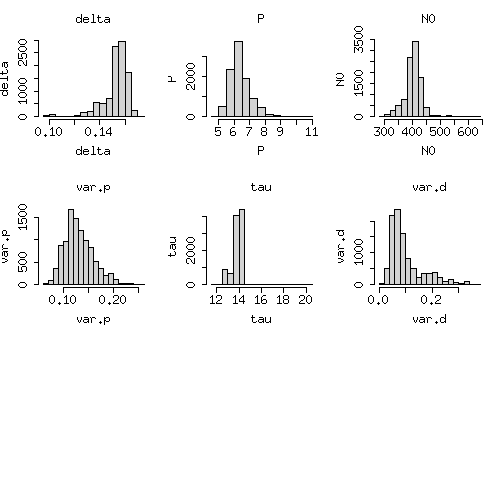## Press <Enter> to see the next plot... ##  "Plotting the log-likelihood chain"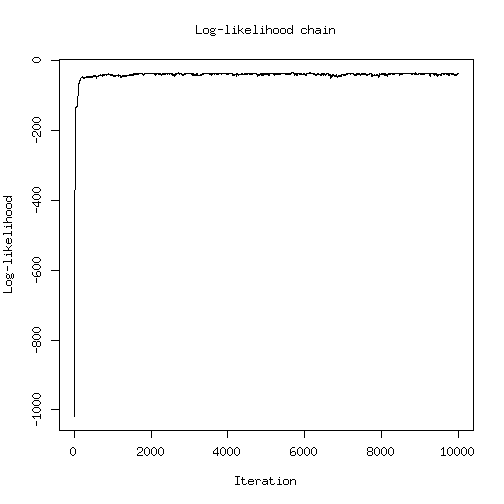## Press <Enter> to see the next plot... ##  "Plotting correlation structure of the posterior sample"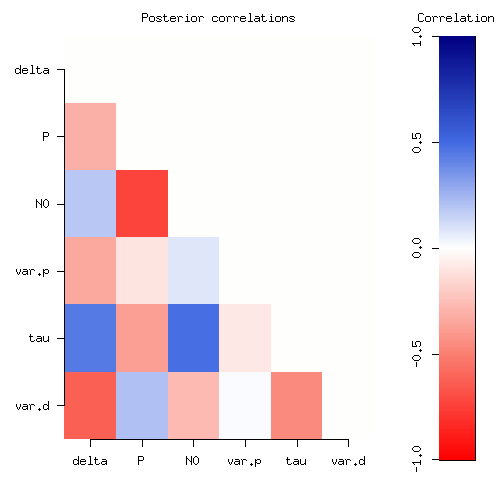## Press <Enter> to see the next plot...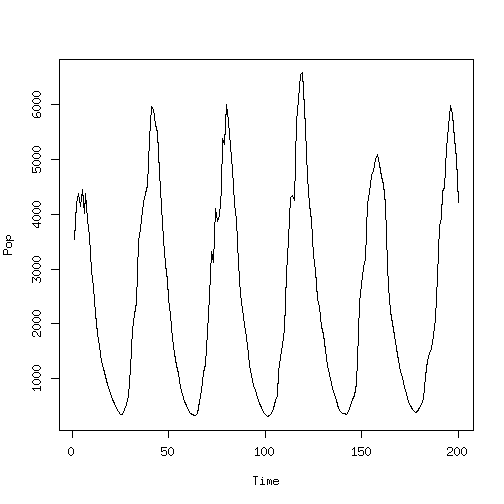The package includes some of Nicholson (1954) experimental datasets, which can be loaded into the object: data(bf1) blow_sl@data <- bf1$pop
blow_sl@extraArgs$obsData <- blow_sl@data  Then it is possible to fit the model using the experimental data by MCMC. ## Alpha-stable distribution Let us consider a model that does not produce time series data: the alpha-stable distribution, as described in Nolan (2012). For a quick reference do library("stableDist") and ?rstable. As a first step we need to wrap the function rstable, which simulates random variables from this distribution, into a function that fits the synlik framework: stableSimul <- function(param, nsim, extraArgs, ...) { if( !is.loaded("stabledist") ) library(stabledist) # Some sanity check if( !( c("nObs") %in% names(extraArgs) ) ) stop("extraArgs should contain nObs") nObs <- extraArgs$nObs
stopifnot( length(param) == 4 )
param[c(1, 3)] <- exp(param[c(1, 3)])
if(abs(param - 1) < 0.01) stop("alpha == 1 is not allowed")

# Actual simulation
output <- rstable(nObs * nsim, alpha = param, beta = param, gamma = param, delta = param)

return( matrix(output, nsim, nObs) )
}


We need also to set up a function that calculates the summary statistics, which in our case are 10 quantiles:

stableStats <- function(x, extraArgs, ...){

if (!is.matrix(x)) x <- matrix(x, 1, length(x))

X0 <- t( apply(x, 1, quantile, probs = seq(0.1, 0.9, length.out = 10)) )

unname(X0)
}


We then create a synlik object:

stable_sl <- synlik( simulator = stableSimul,
summaries = stableStats,
param = c(alpha = log(1.5), beta = 0.1, gamma = log(1), delta = 2),
extraArgs = list("nObs" = 1000),
plotFun = function(input, ...) hist(input, xlab = "x", main = "The data", ...)
)
stable_sl@data <- simulate(stable_sl, seed = 67)
plot(stable_sl)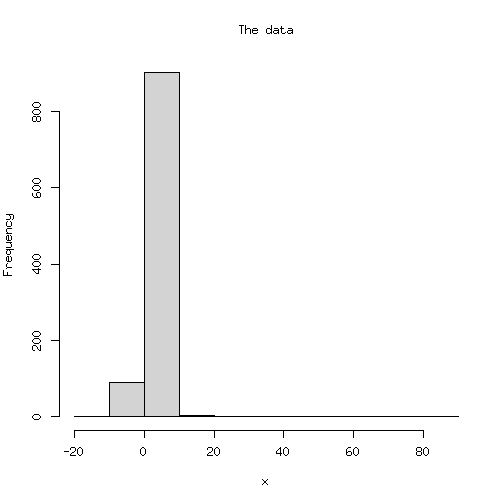As we see from the histogram, the distribution is quite fat-tailed. As before, can then estimate the parameters by MCMC (you might want to increase niter):

stable_sl <- smcmc(stable_sl,
initPar = c(alpha = log(1.7), beta = -0.1, gamma = log(1.3), delta = 1.5),
niter = 2,
burn = 0,
priorFun = function(input, ...) {
dunif(input, log(1), log(2), log = TRUE) +
dunif(input, -1, 1, log = TRUE)
},
propCov = diag(c(0.1, 0.1, 0.1, 0.1))^2,
targetRate = 0.25,
nsim = 200)
# plot(stable_sl, trans = c("alpha" = "exp", "gamma" = "exp"))


By slicing the likelihood we check whether the model is well identified:

slice(object = stable_sl,
ranges = list("alpha" = log(seq(1.2, 1.9, by = 0.05)),
"beta"  = seq(-0.5, 0.5, by = 0.05),
"gamma" = log(seq(0.5, 1.9, by = 0.05)),
"alpha" = seq(1.1, 2.2, by = 0.05)),
param = stable_sl@param,
trans = list("alpha" = "exp", "gamma" = "exp"),
nsim = 1000,
multicore = TRUE,
ncores = 2)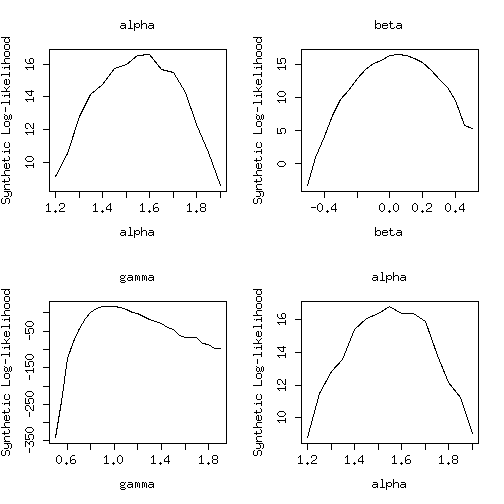We probably could do better by putting some more effort in choosing the summary statistics.

## References

• Simon N Wood. Statistical inference for noisy nonlinear ecological dynamic systems. Nature, 466(7310):1102–1104, 2010.

• Alexander J Nicholson. An outline of the dynamics of animal populations. Australian Journal of Zoology, 2(1):9–65, 1954.

• Diethelm Wuertz, Martin Maechler and Rmetrics core team members. (2013). stabledist: Stable Distribution Functions. R package version 0.6–6.

h